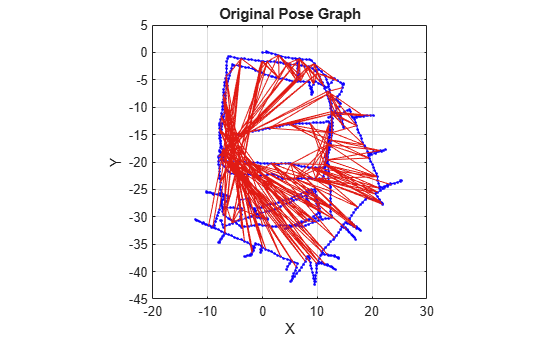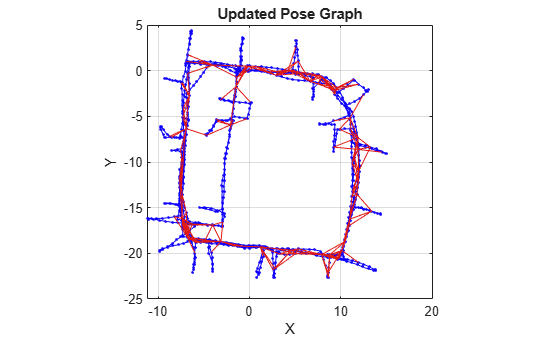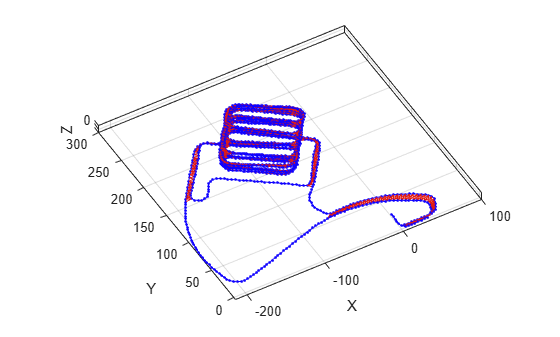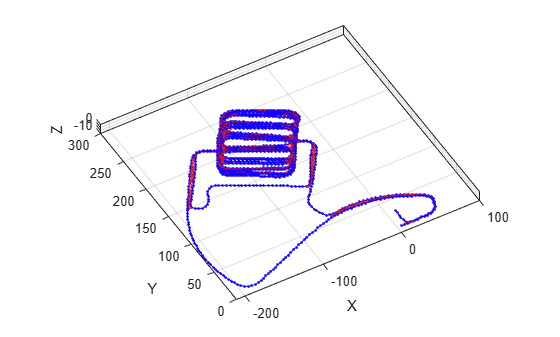# show

Plot pose graph

## Syntax

``show(poseGraph)``
``show(poseGraph,Name,Value)``
``axes = show(___)``

## Description

example

````show(poseGraph)` plots the specified pose graph in a figure.```
````show(poseGraph,Name,Value)` specifies options using `Name,Value` pair arguments. For example, `'IDs','on'` plots all node and edge IDs of the pose graph.```
````axes = show(___)` returns the axes handle that the pose graph is plotted to using any of previous syntaxes.```

## Examples

collapse all

Optimize a pose graph based on the nodes and edge constraints. The pose graph used in this example is from the Intel Research Lab Dataset and was generated from collecting wheel odometry and a laser range finder sensor information in an indoor lab.

Load the Intel data set that contains a 2-D pose graph. Inspect the `poseGraph` object to view the number of nodes and loop closures.

```load intel-2d-posegraph.mat pg disp(pg)```
``` poseGraph with properties: NumNodes: 1228 NumEdges: 1483 NumLoopClosureEdges: 256 LoopClosureEdgeIDs: [1228 1229 1230 1231 1232 1233 1234 1235 1236 ... ] LandmarkNodeIDs: [1x0 double] ```

Plot the pose graph with IDs off. Red lines indicate loop closures identified in the dataset.

```show(pg,'IDs','off'); title('Original Pose Graph')```Optimize the pose graph. Nodes are adjusted based on the edge constraints and loop closures. Plot the optimized pose graph to see the adjustment of the nodes with loop closures.

```updatedPG = optimizePoseGraph(pg); figure show(updatedPG,'IDs','off'); title('Updated Pose Graph')```Optimize a pose graph based on the nodes and edge constraints. The pose graph used in this example is taken from the MIT Dataset and was generated using information extracted from a parking garage.

Load the pose graph from the MIT dataset. Inspect the `poseGraph3D` object to view the number of nodes and loop closures.

```load parking-garage-posegraph.mat pg disp(pg);```
``` poseGraph3D with properties: NumNodes: 1661 NumEdges: 6275 NumLoopClosureEdges: 4615 LoopClosureEdgeIDs: [128 129 130 132 133 134 135 137 138 139 140 ... ] LandmarkNodeIDs: [1x0 double] ```

Plot the pose graph with IDs off. Red lines indicate loop closures identified in the dataset.

```title('Original Pose Graph') show(pg,'IDs','off'); view(-30,45)```Optimize the pose graph. Nodes are adjusted based on the edge constraints and loop closures. Plot the optimized pose graph to see the adjustment of the nodes with loop closures.

```updatedPG = optimizePoseGraph(pg); figure title('Updated Pose Graph') show(updatedPG,'IDs','off'); view(-30,45)```## Input Arguments

collapse all

Pose graph, specified as a `poseGraph` or `poseGraph3D` object.

### Name-Value Arguments

Specify optional comma-separated pairs of `Name,Value` arguments. `Name` is the argument name and `Value` is the corresponding value. `Name` must appear inside quotes. You can specify several name and value pair arguments in any order as `Name1,Value1,...,NameN,ValueN`.

Example: `'IDs','off'`

Axes used to plot the pose graph, specified as the comma-separated pair consisting of `'Parent'` and either an `Axes` or `UIAxes`object. See `axes` or `uiaxes`.

Display of IDs on pose graph, specified as the comma-separated pair consisting of `'IDs'` and one of the following:

• `'all'` — Plot all node and edge IDs.

• `'nodes'` — Plot all node IDs and loop closure IDs.

• `'loopclosures'` — Plot only loop closure edge IDs.

• `'landmarks'` — Plot landmark edge IDs.

• `'off'` — Do not plot any IDs.

## Output Arguments

collapse all

Axes used to plot the map, returned as either an `Axes` or `UIAxes` object. See `axes` or `uiaxes`.